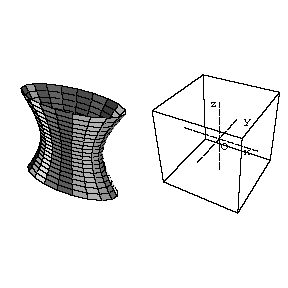# Hyperboloid

(Redirected from One-sheet hyperboloid)

A non-closed central surface of the second order. One distinguishes between two types of hyperboloids: the one-sheet and the two-sheet hyperboloid.Figure: h048360aFigure: h048360b

In a suitable coordinate system (see Fig. a, Fig. b) the equation of a one-sheet hyperboloid is

$$\frac{x^2}{a^2}+\frac{y^2}{b^2}-\frac{z^2}{c^2}=1,$$

while that of a two-sheet hyperboloid is

$$-\frac{x^2}{a^2}-\frac{y^2}{b^2}+\frac{z^2}{c^2}=1.$$

The numbers $a$, $b$ and $c$ (and segments of such lengths) are known as the semi-axes of the hyperboloid. Sections of a hyperboloid by planes passing through the $Oz$-axis are hyperbolas. Sections of a hyperboloid by planes perpendicular to the $Oz$-axis are ellipses. The section of a one-sheet hyperboloid by the plane $z=0$ is said to be a gorge ellipse. A hyperboloid has three planes of symmetry. The cone defined by the equation

$$\frac{x^2}{a^2}+\frac{y^2}{b^2}-\frac{z^2}{c^2}=0$$

is called the asymptotic cone. If $a=b=c$, the hyperboloid is said to be regular. A hyperboloid with two equal semi-axes is said to be a hyperboloid of rotation. A one-sheet hyperboloid is a ruled surface; the equations of the rectilinear generators passing through a given point $(x_0,y_0,z_0)$ have the form

$$\frac{x-x_0}{\frac{ay_0}b}=\frac{y-y_0}{\frac{-bx_0}a}=\frac{z-z_0}{c},$$

$$\frac{x-x_0}{\frac{-ay_0}b}=\frac{y-y_0}{\frac{bx_0}{a}}=\frac{z-z_0}{c}.$$